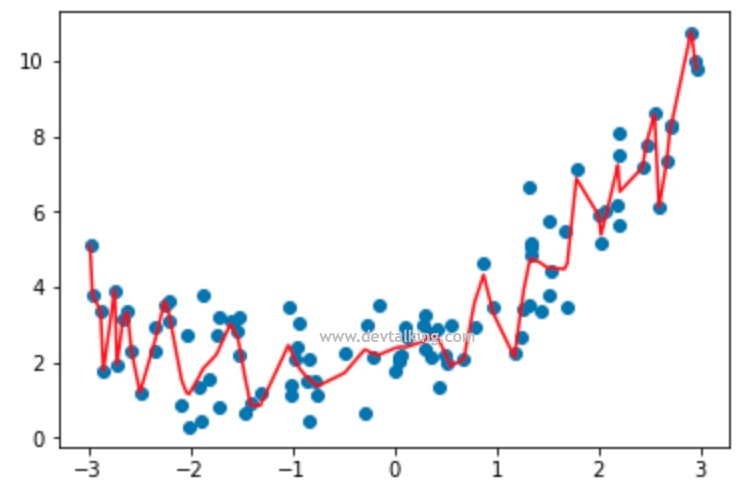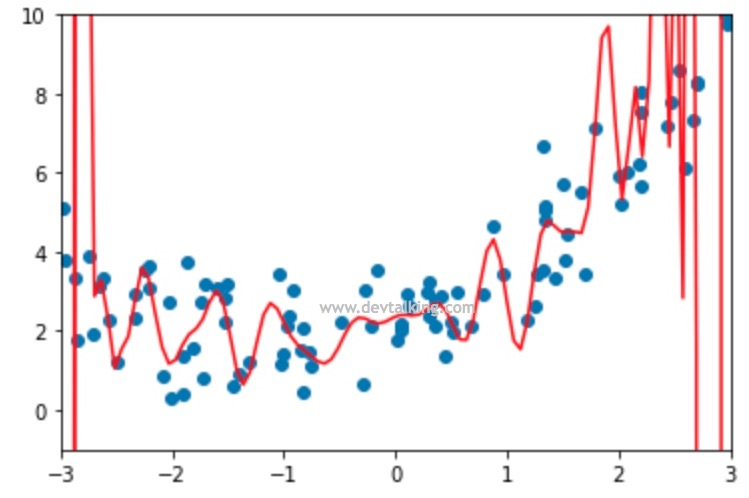## 多项式回归$$y=ax + b$$

$x$就是数据的已知特征，$a$和$b$是我们的模型需要求出的参数。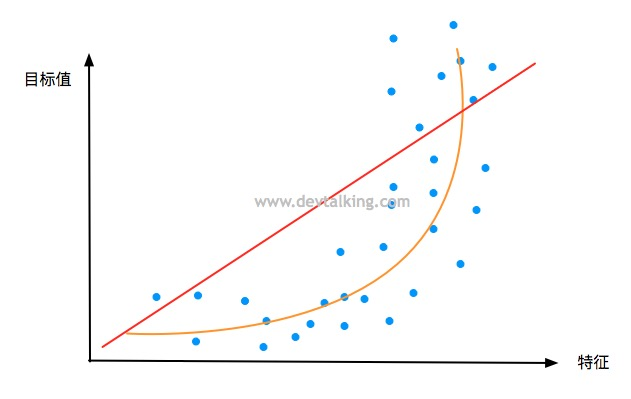$$y = ax^2+bx+c$$

$$y=ax_1+bx_2+c$$

### 实现多项式回归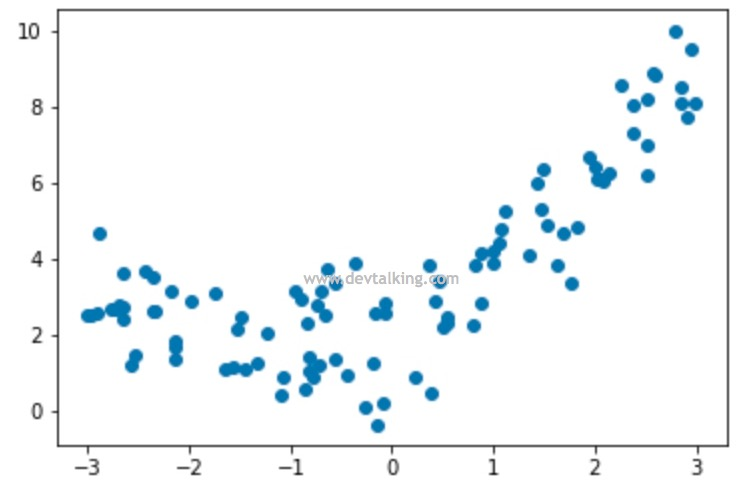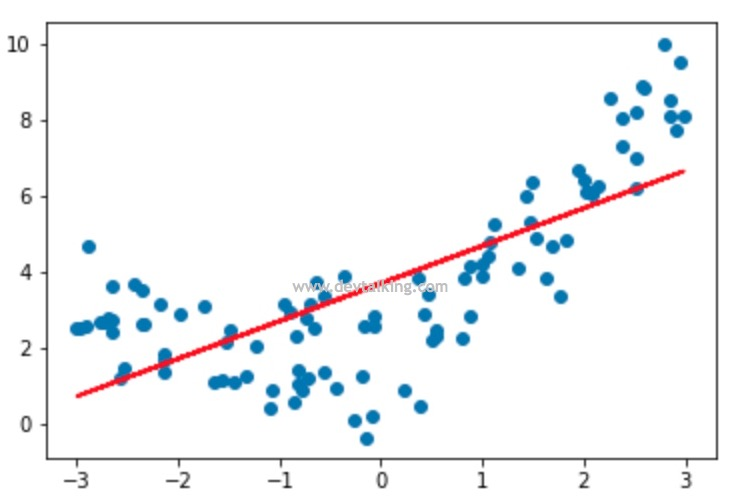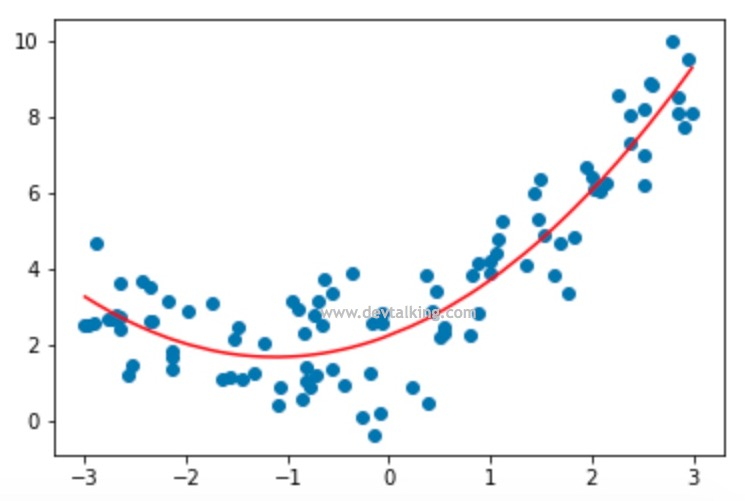## Scikit Learn中的多项式回归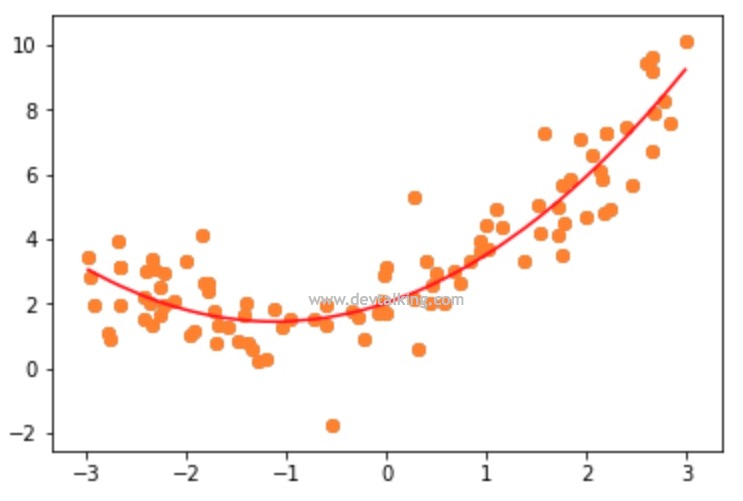• 第一列全部为1。
• 第二列和第三列是原始特征。
• 第四列和第六列分别是第二列原始特征和第三列原始特征的平方。
• 第五列是第二列原始特征和第三列原始特征的乘积。

### Pipeline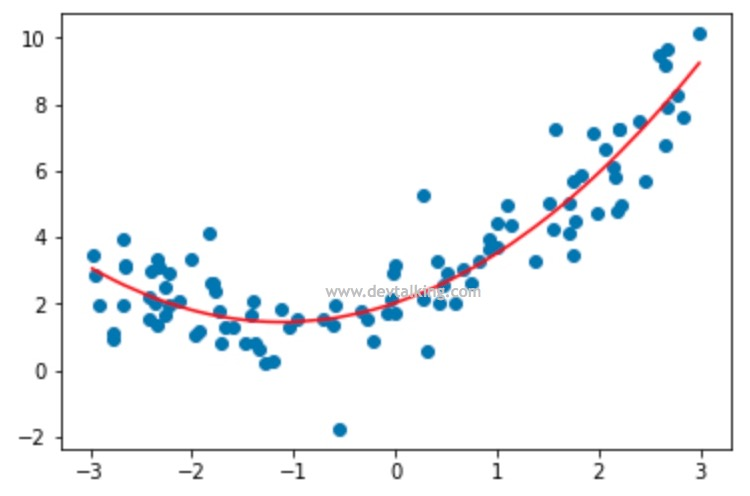## 欠拟合和过拟合

$$\frac 1 m \sum_{i=1}^m(y_{test}^{(i)} - \hat y_{test}^{(i)})^2$$